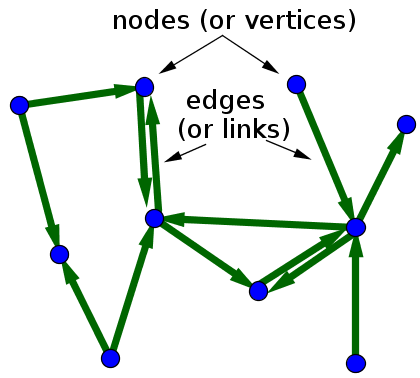# Math Insight

### Directed graph definition

A directed graph is graph, i.e., a set of objects (called vertices or nodes) that are connected together, where all the edges are directed from one vertex to another. A directed graph is sometimes called a digraph or a directed network. In contrast, a graph where the edges are bidirectional is called an undirected graph.

When drawing a directed graph, the edges are typically drawn as arrows indicating the direction, as illustrated in the following figure.A directed graph with 10 vertices (or nodes) and 13 edges.

One can formally define a directed graph as $G= (\mathcal{N},\mathcal{E})$, consisting of the set $\mathcal{N}$ of nodes and the set $\mathcal{E}$ of edges, which are ordered pairs of elements of $\mathcal{N}$.